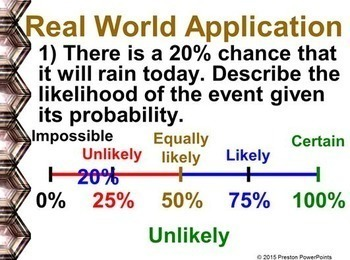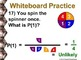(7th) Probability in a PowerPoint PresentationSubject
Resource Type
Product Rating
4.0
2 Ratings
File Type

Compressed Zip File

4 MB|*36
Share
Product Description

7th Grade Math Probability in a PowerPoint Presentation

This slideshow lesson is very animated with a flow-through technique. I developed the lesson for my 7th grade class, Pre-Pre-Algebra, but it can also be used for upper level class reviews. This lesson teaches how to find the probability of an event, and understand the concept of probability and the relationship between probability and likelihood (Certain, likely, unlikely, equally likely or impossible).

This lesson has SKELETON NOTES, notes that have the problem only. This will allow for the students to follow the lesson easier. There are 6 slides per page. They are in a pdf form for easy printing. I also attached the Word document for you to EDIT. If you won’t be doing all of the problems you can shorten what you print off for the skeleton notes.

NEW: The lesson is in an editable format so you can tailor the lesson to your class. The problems and clipart can’t be edited due to the TOU and to maintain the copyright integrity of the product. If you need an alternative version because your country uses different measurements, units, or slight wording adjustment for language differences just email me at PrestonPowerPoints@gmail.com. I am respond to email quickly.

The presentation has 36 slides with LOTS of whiteboard practice. Use as many or as few of the problems to help your students learn each concept. For more PowerPoint lessons & materials visit Preston PowerPoints.

Students often get lost in multi-step math problems. This PowerPoint lesson is unique because it uses a flow-through technique, guided animation, that helps to eliminate confusion and guides the student through the problem. The lesson highlights each step of the problem as the teacher is discussing it, and then animates it to the next step within the lesson. Every step of every problem is shown, even the minor or seemingly insignificant steps. A helpful color-coding technique engages the students and guides them through the problem (Green is for the answer, red for wrong or canceled numbers, & blue, purple & sometimes orange for focusing the next step or separating things.) Twice as many examples are provided, compared to a standard textbook. All lessons have a real-world example to aid the students in visualizing a practical application of the concept.

This lesson applies to the Common Core Standard:

Statistics & Probability 7.SP

Investigate chance processes and develop, use, and evaluate probability models.

5. Understand that the probability of a chance event is a number between 0 and 1 that expresses the likelihood of the event occurring. Larger numbers indicate greater likelihood. A probability near 0 indicates an unlikely event, a probability around 1/2 indicates an event that is neither unlikely nor likely, and a probability near 1 indicates a likely event.

7.Develop a probability model and use it to find probabilities of events. Compare probabilities from a model to observed frequencies; if the agreement is not good, explain possible sources of the discrepancy.

a. Develop a uniform probability model by assigning equal probability to all outcomes, and use the model to determine probabilities of events. For example, if a student is selected at random from a class, find the probability that Jane will be selected and the probability that a girl will be selected.

This resource is for one teacher only. You may not upload this resource to the internet in any form. Additional teachers must purchase their own license. If you are a coach, principal or district interested in purchasing several licenses, please contact me for a district-wide quote at prestonpowerpoints@gmail.com. This product may not be uploaded to the internet in any form, including classroom/personal websites or network drives.

*This lesson contains 28 problems. Each problem in this lesson uses several pages in order to achieve the animated flow-through technique.

Total Pages
*36